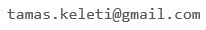﻿ BSM

## Conjecture and Proof

• Instructor: Tamas Keleti
• Contact:• Prerequisites: Introductory math courses, strong problem solving skills
• Text: M. Laczkovich: Conjecture and Proof, Typotex, 1998 (avaliable in digital format, too) and handouts

Description: We try to follow the instruction given by Paul Erdős: Conjecture and prove! According to his spirit, the course intends to show the many and often surprising interrelations between the various branches of mathematics (algebra, analysis, combinatorics, geometry, number theory and set theory) and also to exhibit several unexpected mathematical phenomena via the active problem solving of the students.

This means that instead of following lectures, you need to spend most of your time by problem solving, producing presentations of your solutions, checking and discussing the solutions of the others and learning from them. Most of these activities are between the regular meetings. We will have a tight weekly schedule with deadlines.

A large part of the problems are pretty challenging, this is why strong problem solving skills is needed.

Among others we plan to find answers to the following questions:

• Can the real function f(x)=x be written as the sum of (finitely many) periodic functions?
• Is there a power of 2 whose first 2020 digits are all 7?
• Can you "cut" a ball into finitely many (not necessarily "nice") subsets and "reassemble" two(!) copies of the original ball?
• Can you tile a rectangle of size 1 x √2 with squares?
• Can you construct two subsets of the plane so that their union is congruent (in the usual geometric sense) to both subsets?
Topics:

Invariants for proving impossibility, applications in combinatorics and number theory

Irrational, algebraic and transcendental numbers, their relations to approximation by rationals

Vector spaces, Hamel bases, Cauchy's functional equation, applications

Countable sets, cardinalities, applications of Axiom of Choice and Zorn's Lemma

Isometries of the plane and the space

Isometries, geometric and paradoxical decompositions: Bolyai--Gerwien theorem, Hilbert's third problem, Banach--Tarski paradox

Finite and infinite games, winning strategies

Click here for the first problem set to be discussed. It is posted so that you have a better understanding of this course.